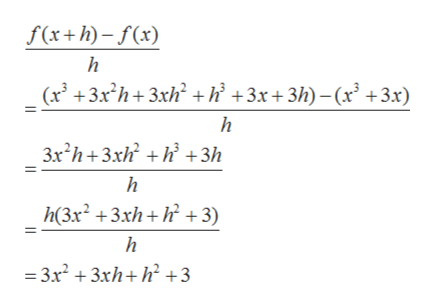# Find the indicated difference quotient and simplify your answer.f (x) = x 3 +3x, f (x +h)− f (x) /h , h does not equal 0 f (x) = 1 /x2 , g (x)− g (3) /x −3 , x does not equal 3.f (t) = 1 / t −2 , f (t)− f (1)/ t −1 , t does not equal 1.

Question
419 views

f (x) = x 3 +3x, f (x +h)− f (x) /h , h does not equal 0

f (x) = 1 /x2 , g (x)− g (3) /x −3 , x does not equal 3.

f (t) = 1 / t −2 , f (t)− f (1)/ t −1 , t does not equal 1.

check_circle

Step 1

Using given f(x) first find f(x+h).

Step 2

Plugged the expression of f(x) and f(x+h) in the difference quotient and simplified. [h does not equal 0]help_outlineImage Transcriptionclosef(x+h)-f(x) h (x3 +3xh+3xh +h'+3x+ 3h)-(x +3x) 3x2h+3xhh3h h h(3x2 3xh h3) h = 3x2 +3xh+h 3 fullscreen
Step 3

Using given g(x) fir...

### Want to see the full answer?

See Solution

#### Want to see this answer and more?

Solutions are written by subject experts who are available 24/7. Questions are typically answered within 1 hour.*

See Solution
*Response times may vary by subject and question.
Tagged in

### Algebra